# Probability Flashcards

## Tags: Mathematics, Probability

Need to master Probability? Use our adaptive flashcards, including all the practice tests and quizzes to learn fast in a short time!

### Top Probability Flashcards Ranked by Quality

• Probability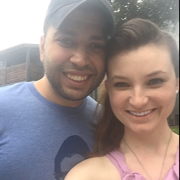Show Class
• Probability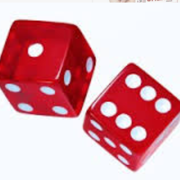Show Class
• Probability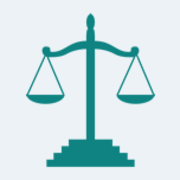Show Class
• ProbabilityShow Class
• Probability & StatisticsShow Class
• Quant - ProbabilityShow Class
• ProbabilityShow Class
• ProbabilityShow Class
• Applied Probability (MAS371)Show Class
• Poker Odds Outs & ProbabilitiesShow Class
• ProbabilityShow Class
• probabilityShow Class
• Poker Math & ProbabilitiesShow Class
• Probability and Distribution TheoryShow Class
• Master List: Statistics and ProbabilityShow Class
• zQuant - ProbabilityShow Class
• Probability modellingShow Class
• Probability & StatsShow Class
• Probability & StatisticsShow Class
• Probability and Statistics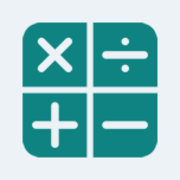Show Class
• Probability and StatisticsShow Class
• Statistics and ProbabilityShow Class
• ProbabilityShow Class
• Intro. To Probability ModelsShow Class
• Probability & StatisticsShow Class
• unit 5 data - probability distributionShow Class
• Applied Probability & Statistics - C955Show Class
• Statistics and Probability Full CourseShow Class
• ProbabilityShow Class
• Statistics, Data Analysis, Probability, & Math ReasoningShow Class
• Intro To Probability And DataShow Class
• Probability & Statistics (Probability Theory)Show Class
• Probability and Distribution TheoryShow Class
• Probability & StatisticsShow Class
• Probability & DistributionsShow Class
• QE Intro Probability and StatisticsShow Class
• GRE Prep- Counting Methods and ProbabilityShow Class
• Probability and StatisticsShow Class
• MATH1712 Probability and Statistics IIShow Class
• Statistics & ProbabilityShow Class
• Probability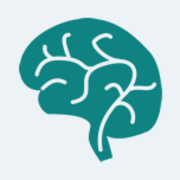Show Class
• Words I should probably knowShow Class
• Probability + Statistics.Show Class
• Statistics & ProbabilityShow Class
• MAS2905:Probability & Bayesian Methods with RShow Class
• Probability And StatisticsShow Class
• Vocab I should probably knowShow Class
• Probability & Statistics 144Show Class
• Fall 2011 - Econ 135Show Class
• zProbability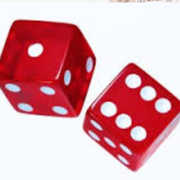Show Class